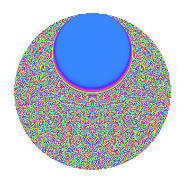# Properties

 Label 4.36.a.aLevel 4 Weight 36 Character orbit 4.a Self dual Yes Analytic conductor 31.038 Analytic rank 1 Dimension 3 CM No Inner twists 1

# Related objects

## Newspace parameters

 Level: $$N$$ = $$4 = 2^{2}$$ Weight: $$k$$ = $$36$$ Character orbit: $$[\chi]$$ = 4.a (trivial)

## Newform invariants

 Self dual: Yes Analytic conductor: $$31.0380522535$$ Analytic rank: $$1$$ Dimension: $$3$$ Coefficient field: $$\mathbb{Q}[x]/(x^{3} - \cdots)$$ Coefficient ring: $$\Z[a_1, \ldots, a_{5}]$$ Coefficient ring index: $$2^{24}\cdot 3^{4}\cdot 5\cdot 7$$ Fricke sign: $$-1$$ Sato-Tate group: $\mathrm{SU}(2)$

## $q$-expansion

Coefficients of the $$q$$-expansion are expressed in terms of a basis $$1,\beta_1,\beta_2$$ for the coefficient ring described below. We also show the integral $$q$$-expansion of the trace form.

 $$f(q)$$ $$=$$ $$q$$ $$+ ( 16969628 + \beta_{1} ) q^{3}$$ $$+ ( 93573630 + 246 \beta_{1} - \beta_{2} ) q^{5}$$ $$+ ( -1849765429672 - 244638 \beta_{1} + 564 \beta_{2} ) q^{7}$$ $$+ ( -1371898039365771 - 77158812 \beta_{1} - 15318 \beta_{2} ) q^{9}$$ $$+O(q^{10})$$ $$q$$ $$+(16969628 + \beta_{1}) q^{3}$$ $$+(93573630 + 246 \beta_{1} - \beta_{2}) q^{5}$$ $$+(-1849765429672 - 244638 \beta_{1} + 564 \beta_{2}) q^{7}$$ $$+(-1371898039365771 - 77158812 \beta_{1} - 15318 \beta_{2}) q^{9}$$ $$+(-140154652956383820 - 8262293541 \beta_{1} + 17576 \beta_{2}) q^{11}$$ $$+(-2456900438471578858 - 145960301034 \beta_{1} + 5745423 \beta_{2}) q^{13}$$ $$+(11912600874497874120 + 750084516054 \beta_{1} - 131835924 \beta_{2}) q^{15}$$ $$+($$$$20\!\cdots\!86$$$$+ 12417058928292 \beta_{1} + 1633047370 \beta_{2}) q^{17}$$ $$+(-$$$$11\!\cdots\!16$$$$- 48219784860123 \beta_{1} - 13362439272 \beta_{2}) q^{19}$$ $$+(-$$$$11\!\cdots\!24$$$$- 414877019931448 \beta_{1} + 75977545428 \beta_{2}) q^{21}$$ $$+(-$$$$32\!\cdots\!24$$$$+ 1845485459987046 \beta_{1} - 296037672404 \beta_{2}) q^{23}$$ $$+(-$$$$46\!\cdots\!25$$$$+ 5981927839966440 \beta_{1} + 717372157860 \beta_{2}) q^{25}$$ $$+(-$$$$46\!\cdots\!24$$$$- 32297545853297046 \beta_{1} - 779822285112 \beta_{2}) q^{27}$$ $$+(-$$$$24\!\cdots\!66$$$$- 56580793878865506 \beta_{1} + 857835418691 \beta_{2}) q^{29}$$ $$+(-$$$$10\!\cdots\!44$$$$+ 334440004372376040 \beta_{1} - 18744137713440 \beta_{2}) q^{31}$$ $$+(-$$$$40\!\cdots\!80$$$$+ 623973325316463756 \beta_{1} + 128812730285934 \beta_{2}) q^{33}$$ $$+(-$$$$13\!\cdots\!80$$$$- 3453236753480364756 \beta_{1} - 390637255582064 \beta_{2}) q^{35}$$ $$+(-$$$$34\!\cdots\!62$$$$- 1297334320812122562 \beta_{1} + 198623459819283 \beta_{2}) q^{37}$$ $$+(-$$$$71\!\cdots\!16$$$$+ 6840061094178693230 \beta_{1} + 2971622977394220 \beta_{2}) q^{39}$$ $$+(-$$$$95\!\cdots\!34$$$$+ 63123754486312617384 \beta_{1} - 11777845226841724 \beta_{2}) q^{41}$$ $$+(-$$$$68\!\cdots\!00$$$$-$$$$17\!\cdots\!37$$$$\beta_{1} + 16341886387601712 \beta_{2}) q^{43}$$ $$+($$$$36\!\cdots\!70$$$$+ 30929043727996135974 \beta_{1} + 21657827445373431 \beta_{2}) q^{45}$$ $$+($$$$14\!\cdots\!76$$$$-$$$$23\!\cdots\!60$$$$\beta_{1} - 133055352405484248 \beta_{2}) q^{47}$$ $$+($$$$40\!\cdots\!73$$$$+$$$$19\!\cdots\!56$$$$\beta_{1} + 212273841375916584 \beta_{2}) q^{49}$$ $$+($$$$60\!\cdots\!32$$$$-$$$$22\!\cdots\!74$$$$\beta_{1} + 18936105471182664 \beta_{2}) q^{51}$$ $$+($$$$69\!\cdots\!14$$$$-$$$$76\!\cdots\!18$$$$\beta_{1} - 627221916303572269 \beta_{2}) q^{53}$$ $$+(-$$$$14\!\cdots\!00$$$$-$$$$62\!\cdots\!50$$$$\beta_{1} + 1076106883834559700 \beta_{2}) q^{55}$$ $$+(-$$$$23\!\cdots\!08$$$$+$$$$13\!\cdots\!12$$$$\beta_{1} - 972666146017593198 \beta_{2}) q^{57}$$ $$+(-$$$$72\!\cdots\!92$$$$+$$$$24\!\cdots\!59$$$$\beta_{1} + 582701521780060576 \beta_{2}) q^{59}$$ $$+(-$$$$15\!\cdots\!18$$$$-$$$$41\!\cdots\!58$$$$\beta_{1} + 2399951807059995063 \beta_{2}) q^{61}$$ $$+(-$$$$20\!\cdots\!28$$$$-$$$$19\!\cdots\!30$$$$\beta_{1} - 12132436053826620396 \beta_{2}) q^{63}$$ $$+(-$$$$15\!\cdots\!20$$$$-$$$$14\!\cdots\!64$$$$\beta_{1} + 14938326422972709484 \beta_{2}) q^{65}$$ $$+($$$$25\!\cdots\!28$$$$+$$$$37\!\cdots\!93$$$$\beta_{1} + 22709891622220915608 \beta_{2}) q^{67}$$ $$+($$$$88\!\cdots\!72$$$$+$$$$22\!\cdots\!92$$$$\beta_{1} - 66182008910064631812 \beta_{2}) q^{69}$$ $$+($$$$19\!\cdots\!48$$$$+$$$$11\!\cdots\!62$$$$\beta_{1} - 29755918607961419132 \beta_{2}) q^{71}$$ $$+($$$$28\!\cdots\!22$$$$-$$$$98\!\cdots\!04$$$$\beta_{1} +$$$$21\!\cdots\!78$$$$\beta_{2}) q^{73}$$ $$+($$$$28\!\cdots\!00$$$$-$$$$15\!\cdots\!65$$$$\beta_{1} + 241028779072562640 \beta_{2}) q^{75}$$ $$+($$$$12\!\cdots\!20$$$$+$$$$34\!\cdots\!56$$$$\beta_{1} -$$$$62\!\cdots\!16$$$$\beta_{2}) q^{77}$$ $$+(-$$$$50\!\cdots\!48$$$$+$$$$14\!\cdots\!84$$$$\beta_{1} +$$$$31\!\cdots\!76$$$$\beta_{2}) q^{79}$$ $$+(-$$$$15\!\cdots\!11$$$$+$$$$28\!\cdots\!68$$$$\beta_{1} +$$$$11\!\cdots\!02$$$$\beta_{2}) q^{81}$$ $$+(-$$$$29\!\cdots\!04$$$$-$$$$10\!\cdots\!35$$$$\beta_{1} -$$$$53\!\cdots\!08$$$$\beta_{2}) q^{83}$$ $$+(-$$$$38\!\cdots\!60$$$$-$$$$15\!\cdots\!52$$$$\beta_{1} -$$$$28\!\cdots\!38$$$$\beta_{2}) q^{85}$$ $$+(-$$$$31\!\cdots\!68$$$$-$$$$19\!\cdots\!50$$$$\beta_{1} +$$$$97\!\cdots\!44$$$$\beta_{2}) q^{87}$$ $$+($$$$54\!\cdots\!66$$$$+$$$$32\!\cdots\!52$$$$\beta_{1} +$$$$84\!\cdots\!78$$$$\beta_{2}) q^{89}$$ $$+($$$$96\!\cdots\!44$$$$+$$$$79\!\cdots\!56$$$$\beta_{1} -$$$$87\!\cdots\!16$$$$\beta_{2}) q^{91}$$ $$+($$$$14\!\cdots\!68$$$$-$$$$11\!\cdots\!84$$$$\beta_{1} -$$$$75\!\cdots\!60$$$$\beta_{2}) q^{93}$$ $$+($$$$32\!\cdots\!20$$$$+$$$$41\!\cdots\!74$$$$\beta_{1} +$$$$16\!\cdots\!56$$$$\beta_{2}) q^{95}$$ $$+($$$$27\!\cdots\!38$$$$-$$$$11\!\cdots\!96$$$$\beta_{1} -$$$$85\!\cdots\!58$$$$\beta_{2}) q^{97}$$ $$+($$$$30\!\cdots\!20$$$$-$$$$14\!\cdots\!09$$$$\beta_{1} +$$$$60\!\cdots\!24$$$$\beta_{2}) q^{99}$$ $$+O(q^{100})$$ $$\operatorname{Tr}(f)(q)$$ $$=$$ $$3q$$ $$\mathstrut +\mathstrut 50908884q^{3}$$ $$\mathstrut +\mathstrut 280720890q^{5}$$ $$\mathstrut -\mathstrut 5549296289016q^{7}$$ $$\mathstrut -\mathstrut 4115694118097313q^{9}$$ $$\mathstrut +\mathstrut O(q^{10})$$ $$3q$$ $$\mathstrut +\mathstrut 50908884q^{3}$$ $$\mathstrut +\mathstrut 280720890q^{5}$$ $$\mathstrut -\mathstrut 5549296289016q^{7}$$ $$\mathstrut -\mathstrut 4115694118097313q^{9}$$ $$\mathstrut -\mathstrut 420463958869151460q^{11}$$ $$\mathstrut -\mathstrut 7370701315414736574q^{13}$$ $$\mathstrut +\mathstrut 35737802623493622360q^{15}$$ $$\mathstrut +\mathstrut$$$$61\!\cdots\!58$$$$q^{17}$$ $$\mathstrut -\mathstrut$$$$35\!\cdots\!48$$$$q^{19}$$ $$\mathstrut -\mathstrut$$$$35\!\cdots\!72$$$$q^{21}$$ $$\mathstrut -\mathstrut$$$$97\!\cdots\!72$$$$q^{23}$$ $$\mathstrut -\mathstrut$$$$13\!\cdots\!75$$$$q^{25}$$ $$\mathstrut -\mathstrut$$$$13\!\cdots\!72$$$$q^{27}$$ $$\mathstrut -\mathstrut$$$$72\!\cdots\!98$$$$q^{29}$$ $$\mathstrut -\mathstrut$$$$30\!\cdots\!32$$$$q^{31}$$ $$\mathstrut -\mathstrut$$$$12\!\cdots\!40$$$$q^{33}$$ $$\mathstrut -\mathstrut$$$$41\!\cdots\!40$$$$q^{35}$$ $$\mathstrut -\mathstrut$$$$10\!\cdots\!86$$$$q^{37}$$ $$\mathstrut -\mathstrut$$$$21\!\cdots\!48$$$$q^{39}$$ $$\mathstrut -\mathstrut$$$$28\!\cdots\!02$$$$q^{41}$$ $$\mathstrut -\mathstrut$$$$20\!\cdots\!00$$$$q^{43}$$ $$\mathstrut +\mathstrut$$$$10\!\cdots\!10$$$$q^{45}$$ $$\mathstrut +\mathstrut$$$$43\!\cdots\!28$$$$q^{47}$$ $$\mathstrut +\mathstrut$$$$12\!\cdots\!19$$$$q^{49}$$ $$\mathstrut +\mathstrut$$$$18\!\cdots\!96$$$$q^{51}$$ $$\mathstrut +\mathstrut$$$$20\!\cdots\!42$$$$q^{53}$$ $$\mathstrut -\mathstrut$$$$42\!\cdots\!00$$$$q^{55}$$ $$\mathstrut -\mathstrut$$$$70\!\cdots\!24$$$$q^{57}$$ $$\mathstrut -\mathstrut$$$$21\!\cdots\!76$$$$q^{59}$$ $$\mathstrut -\mathstrut$$$$46\!\cdots\!54$$$$q^{61}$$ $$\mathstrut -\mathstrut$$$$60\!\cdots\!84$$$$q^{63}$$ $$\mathstrut -\mathstrut$$$$47\!\cdots\!60$$$$q^{65}$$ $$\mathstrut +\mathstrut$$$$75\!\cdots\!84$$$$q^{67}$$ $$\mathstrut +\mathstrut$$$$26\!\cdots\!16$$$$q^{69}$$ $$\mathstrut +\mathstrut$$$$59\!\cdots\!44$$$$q^{71}$$ $$\mathstrut +\mathstrut$$$$84\!\cdots\!66$$$$q^{73}$$ $$\mathstrut +\mathstrut$$$$84\!\cdots\!00$$$$q^{75}$$ $$\mathstrut +\mathstrut$$$$36\!\cdots\!60$$$$q^{77}$$ $$\mathstrut -\mathstrut$$$$15\!\cdots\!44$$$$q^{79}$$ $$\mathstrut -\mathstrut$$$$47\!\cdots\!33$$$$q^{81}$$ $$\mathstrut -\mathstrut$$$$89\!\cdots\!12$$$$q^{83}$$ $$\mathstrut -\mathstrut$$$$11\!\cdots\!80$$$$q^{85}$$ $$\mathstrut -\mathstrut$$$$94\!\cdots\!04$$$$q^{87}$$ $$\mathstrut +\mathstrut$$$$16\!\cdots\!98$$$$q^{89}$$ $$\mathstrut +\mathstrut$$$$28\!\cdots\!32$$$$q^{91}$$ $$\mathstrut +\mathstrut$$$$43\!\cdots\!04$$$$q^{93}$$ $$\mathstrut +\mathstrut$$$$96\!\cdots\!60$$$$q^{95}$$ $$\mathstrut +\mathstrut$$$$83\!\cdots\!14$$$$q^{97}$$ $$\mathstrut +\mathstrut$$$$91\!\cdots\!60$$$$q^{99}$$ $$\mathstrut +\mathstrut O(q^{100})$$

Basis of coefficient ring in terms of a root $$\nu$$ of $$x^{3}\mathstrut -\mathstrut$$ $$x^{2}\mathstrut -\mathstrut$$ $$1597028177$$ $$x\mathstrut +\mathstrut$$ $$23572260890640$$:

 $$\beta_{0}$$ $$=$$ $$1$$ $$\beta_{1}$$ $$=$$ $$($$$$256 \nu^{2} + 4304128 \nu - 272560910336$$$$)/319$$ $$\beta_{2}$$ $$=$$ $$($$$$1491456 \nu^{2} + 44832004608 \nu - 1587946449002496$$$$)/319$$
 $$1$$ $$=$$ $$\beta_0$$ $$\nu$$ $$=$$ $$($$$$\beta_{2}\mathstrut -\mathstrut$$ $$5826$$ $$\beta_{1}\mathstrut +\mathstrut$$ $$20643840$$$$)/61931520$$ $$\nu^{2}$$ $$=$$ $$($$$$-$$$$16813$$ $$\beta_{2}\mathstrut +\mathstrut$$ $$175125018$$ $$\beta_{1}\mathstrut +\mathstrut$$ $$65937588343603200$$$$)/61931520$$

## Embeddings

For each embedding $$\iota_m$$ of the coefficient field, the values $$\iota_m(a_n)$$ are shown below.

For more information on an embedded modular form you can click on its label.

Label $$\iota_m(\nu)$$ $$a_{2}$$ $$a_{3}$$ $$a_{4}$$ $$a_{5}$$ $$a_{6}$$ $$a_{7}$$ $$a_{8}$$ $$a_{9}$$ $$a_{10}$$
1.1
 19173.3 26763.9 −45936.2
0 −2.83743e8 0 4.90658e11 0 −2.46684e14 0 3.04783e16 0
1.2 0 9.85025e7 0 −2.11237e12 0 1.18095e15 0 −4.03288e16 0
1.3 0 2.36149e8 0 1.62199e12 0 −9.39810e14 0 5.73480e15 0
 $$n$$: e.g. 2-40 or 990-1000 Significant digits: Format: Complex embeddings Normalized embeddings Satake parameters Satake angles

## Inner twists

This newform does not admit any (nontrivial) inner twists.

## Atkin-Lehner signs

$$p$$ Sign
$$2$$ $$-1$$

## Hecke kernels

There are no other newforms in $$S_{36}^{\mathrm{new}}(\Gamma_0(4))$$.